Warning

# High Dynamic Range (HDR)

High Dynamic Range (HDR) imaging is a technique for capturing and reproducing a greater dynamic range of luminosity than is possible with standard digital imaging or photographic techniques.

We will consider two basic tasks:

1. Composition of an HDR image from a set of images, each one having a much smaller dynamic range than the resulting HDR image (week 1), and
2. Compression of the HDR for the purpose of its displaying or printing, called tone mapping (week 2).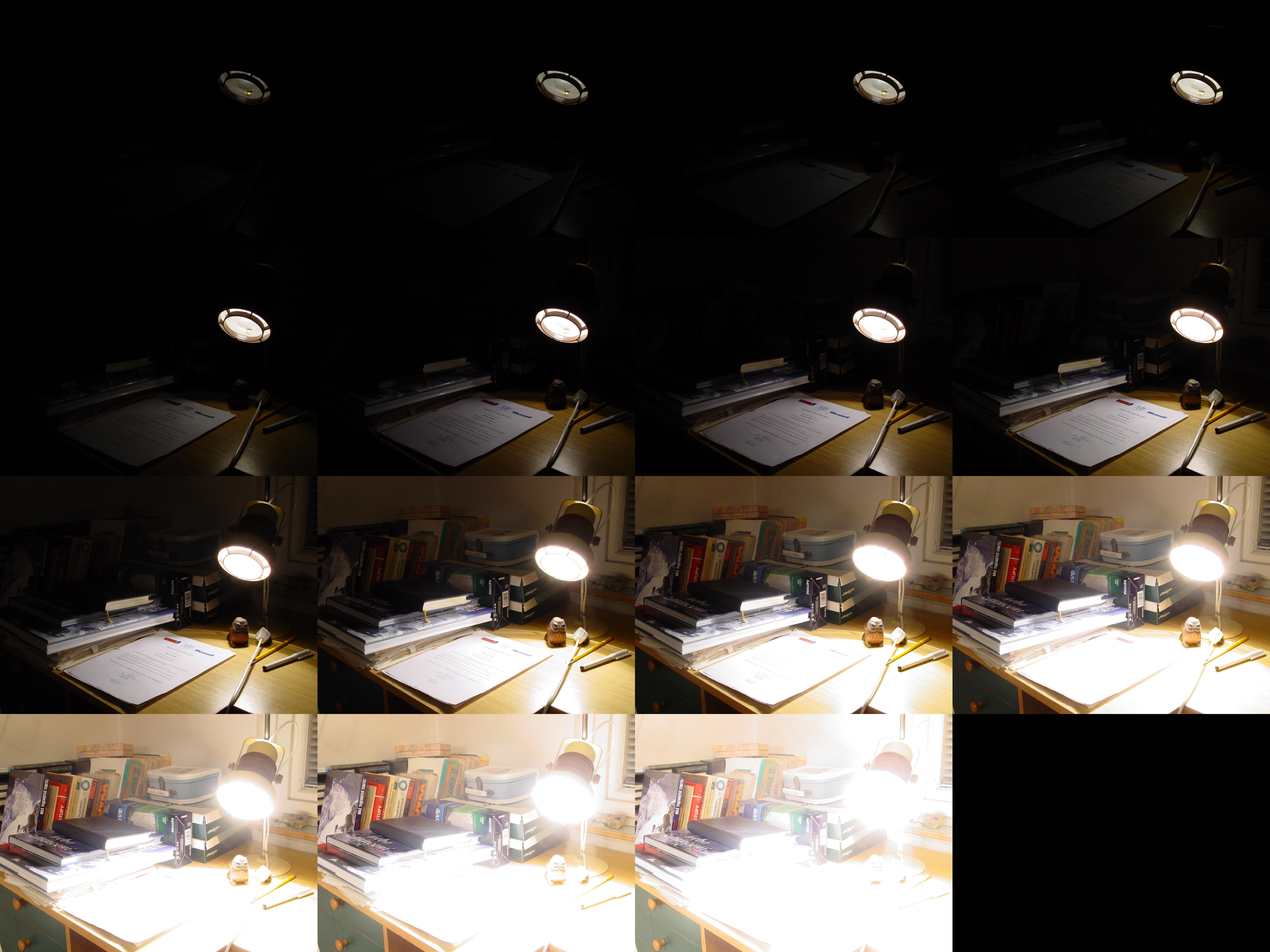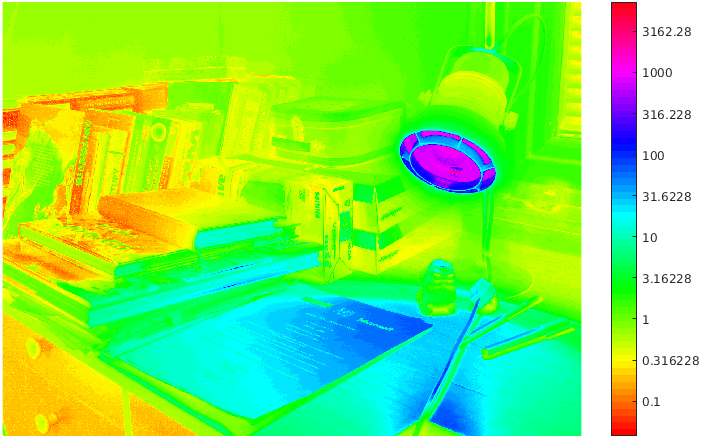Sequence of photographs taken with varying exposure times Reconstructed irradiance map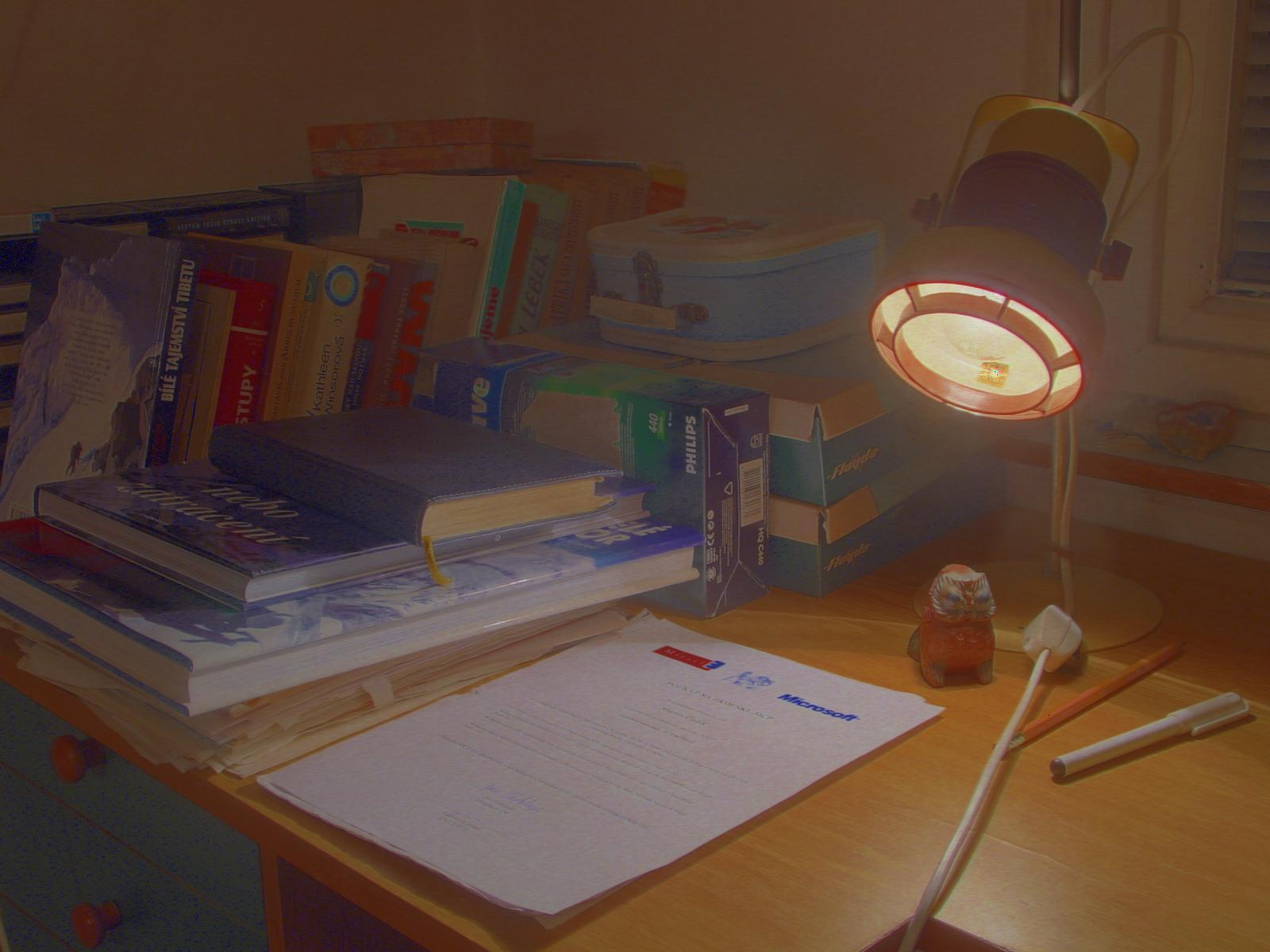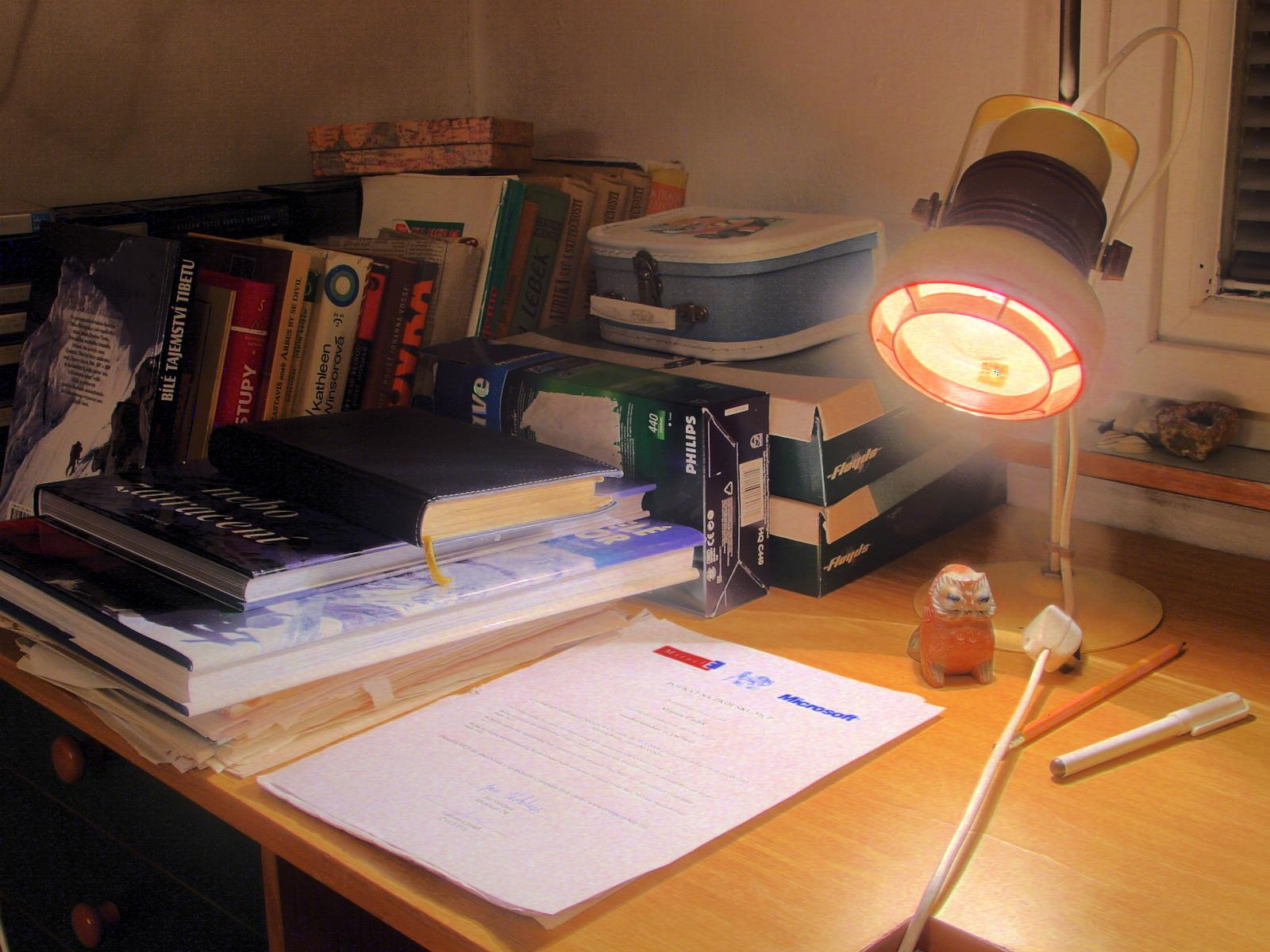HDR image with logarithmic tone mapping HDR image with equalized tone mapping

## Composition of HDR Image

The intensity $Z$ at a particular pixel relates to the irradiance $E$ at the corresponding sensor element and the exposure time $t$ by $Z = f(E t) = f(X)$, where $X = E t$ is called exposure. The mapping $f$ is possibly nonlinear, and can capture a multitude of effects including the response characteristics of the sensor, scanning process, digitization, postprocessing etc.

Note that we cannot in principle distinguish a change of the exposure time from a change of the irradiance from the sensor readings alone since $X = E t = (\alpha E)(t / \alpha)$ for any $\alpha \neq 0$; this property is known as reciprocity.

In composing the HDR image we will consider two different situations, depending on what is assumed to be known.

### Exposure Estimation

In the first case, the mapping $f$ is assumed to be known. We are also given $P$ images with $N$ pixels each. The intensity at pixel $i$ in image $j$ is $Z_{i,j} = f(E_i t_j)$. Our task is to estimate

• irradiance $E_i$ for every pixel $i \in \{1 \ldots N\}$, and
• exposure time $t_j$ for every image $j \in \{1 \ldots P\}$.

Assuming that the given $f$ is strictly monotonic (and therefore invertible), we can derive a set of equations as follows:

\begin{align*} Z_{i,j} &= f(E_i t_j), \\ f^{-1}(Z_{i,j}) &= E_i t_j, \\ \log(f^{-1}(Z_{i,j})) &= \log(E_i) + \log(t_j), \qquad i\in \{1 \ldots N\}, j\in \{1 \ldots P\}. \end{align*}

Let us denote $g(Z_{i,j}) = \log(f^{-1}(Z_{i,j}))$ and rewrite this: \begin{equation} \label{eq-exposure} \log(E_i) + \log(t_j) = g(Z_{i,j}), \qquad i\in \{1 \ldots N\}, j\in \{1 \ldots P\}. \end{equation} All $g(Z_{i,j})$'s are known. The uknowns in this system of equations are $\log(E_i)$'s and $\log(t_j)$'s. The system of equations is linear in these unknowns and can be written in a matrix form as $\mathbf{A} \mathbf{x} = \mathbf{b}$. We can write out the coefficients of matrix $\mathbf{A}$ and vector $\mathbf{b}$ as follows:

\begin{equation} \label{eq-matrix-exposure} \begin{array}{c|c} \mathbf{x}^T & \\\hline \mathbf{A} & \mathbf{b}\\ \end{array} = \begin{array}{cccccccc|c} \log(E_1) & \log(E_2) & \dots & \log(E_N) & \log(t_1) & \log(t_2) & \dots & \log(t_P) \\\hline \color{red}{1} & 0 & \dots & 0 & \color{red}{1} & 0 & \dots & 0 & g(Z_{1,1})\\ 0 & \color{red}{1} & \dots & 0 & \color{red}{1} & 0 & \dots & 0 & g(Z_{2,1})\\ \vdots & \vdots & \ddots & \vdots & \vdots & \vdots & \ddots & \vdots & \vdots\\ 0 & 0 & \dots & \color{red}{1} & \color{red}{1} & 0 & \dots & 0 & g(Z_{N, 1})\\ \color{green}{1} & 0 & \dots & 0 & 0 & \color{green}{1} & \dots & 0 & g(Z_{1,2})\\ 0 & \color{green}{1} & \dots & 0 & 0 & \color{green}{1} & \dots & 0 & g(Z_{2,2})\\ \vdots & \vdots & \ddots & \vdots & \vdots & \vdots & \ddots & \vdots & \vdots\\ 0 & 0 & \dots & \color{green}{1} & 0 & \color{green}{1} & \dots & 0 & g(Z_{N, 2})\\ % \vdots & \vdots & \vdots & \vdots & \vdots & \vdots & \vdots & \vdots & \vdots\\ \vdots & \vdots & \vdots & \vdots & \vdots & \vdots & \vdots & \vdots & \vdots\\ \color{orange}{1} & 0 & \dots & 0 & 0 & 0 & \dots & \color{orange}{1} & g(Z_{1,P})\\ 0 & \color{orange}{1} & \dots & 0 & 0 & 0 & \dots & \color{orange}{1} & g(Z_{2,P})\\ \vdots & \vdots & \ddots & \vdots & \vdots & \vdots & \ddots & \vdots & \vdots\\ 0 & 0 & \dots & \color{orange}{1} & 0 & 0 & \dots & \color{orange}{1} & g(Z_{N, P})\\ \end{array} \end{equation}

Note that most of the matrix coefficients are zeros, i.e., the matrix is sparse.

Generally, the number of equations $N \cdot P$ in this system will be much higher than the number of unknowns $N + P$. The system is therefore overdetermined. It is not possible to find solution satisfying all the equations. However, we can find the solution minimizing the least-squares errors. Each equation will be associated with the following error (squared difference of its right and left side): \begin{equation} \left[ \log(E_i) + \log(t_j) - g(Z_{i,j}) \right]^2 \end{equation}

In addition, we will weight the importance of each equation in the system. We will minimize the following overall error: \begin{equation} \label{eq-response-squares} \sum_{i=1}^N \sum_{j=1}^P w(Z_{i,j}) \left[ \log(E_i) + \log(t_j) - g(Z_{i,j}) \right]^2. \end{equation}

Here $w:\mathbb{N}_0\rightarrow[0, 1]$ is the weight function. The weights $w(Z_{i,j})$ depend only on the pixel intensity $Z_{i,j}$ and represent how much the measurement of and intensity $Z_{i,j}$ is 'trusted'. For example, if $Z_{i,j}=255$ for an 8-bit sensor, the sensor is saturated and the actual sensor reading should be higher. For such case $w$ should be $0$. On the other hand, for lower intensities rounding errors are more pronounced. We limit the influence of such measurements by using the following weight function:

Introducing the weights into our system of linear equations is accomplished by multiplying each row of both $\mathbf{A}$ and $\mathbf{b}$ by the squared root of the corresponding weight $\sqrt{w(Z_{i,j})}$.

Finally, we introduce additional constraint \begin{equation} \label{eq-time-scale} \log(t_1) = 0 \end{equation} to remove the reciprocity ambiguity. This will add one row to $\mathbf{A}$ and one element to $\mathbf{b}$.

To recap, building the system of equations involves:

1. Creating the matrix $\mathbf{A}$ and the vector $\mathbf{b}$, according to (\ref{eq-exposure}) and (\ref{eq-matrix-exposure}), with row values multiplied by square roots of the weights from (\ref{eq-response-squares}): this creates the core of the system of equations.
2. Resolving the ambiguity by adding (\ref{eq-time-scale}): this fixes the rank of the system of equations.

Once the solution $\mathbf{x}$ is found, all $\log(E_i)$'s and $\log(t_j)$'s can be extracted from it. Possible irradiances and exposure times you may obtain: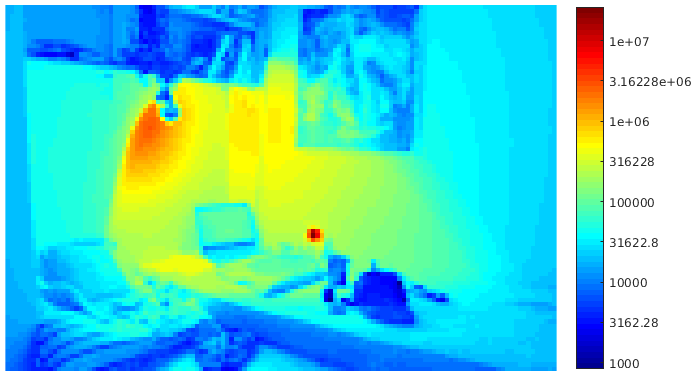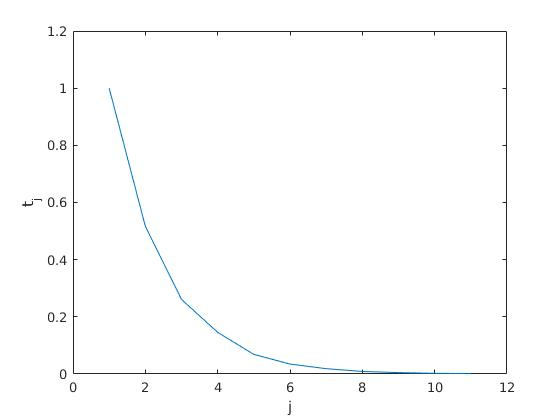### Response Function Estimation

In the second case, the exposure times $t_1, t_2, ..., t_P$ are given. Besides that, $P$ images with $N$ pixels each are given, as in the previous case. Again, the intensity at pixel $i$ in image $j$ is $Z_{i,j} = f(E_i t_j)$. Our task is to estimate

• irradiance $E_i$ for every pixel $i \in \{1 \ldots N\}$, and
• values of the inverse response function $f^{-1}: \{0 \ldots L-1\} \to [0, \infty)$ for all possible sensor intensities $z \in \{0 \ldots L-1\}$. For example, $L=2^8$ for an 8-bit sensor.

This leads to the same equations as in the previous case but with different known and unknown variables. Let us formally rewrite the system such that the knowns are again on the right-hand side: \begin{equation} \label{eq-response} g(Z_{i,j}) - \log(E_i) = \log(t_j), \qquad i\in \{1 \ldots N\}, j\in \{1 \ldots P\}, \end{equation} with $g(Z_{i,j}) = \log f^{-1}(Z_{i,j})$ and unknowns $g(0), \ldots, g(L-1)$ and $\log(E_1), \ldots, \log(E_N)$.

The coefficient matrix $\mathbf{A}$ and the right-hand side vector $\mathbf{b}$ change correspondingly. Let us outline the change on an example. If we know that the intensity $Z_{2,8}=100$ at pixel $i=2$ in image $j=8$, the following equation is added to the system: \begin{equation} \label{eq-matrix-response} \begin{array}{cccccccccc|c} g(0) & g(1) & \dots & g(100) & \dots & g(L-1) & \log E_1 & \log E_2 & \dots & \log E_N & \\\hline 0 & 0 & \dots & \color{red}{1} & \dots & 0 & 0 & \color{red}{-1} & \dots & 0 & \log{t_8}\\ \end{array} \end{equation}

Note that the position of non-zero coefficients in the matrix $\mathbf{A}$ does not only depend on the pixel index $i$ and the image index $j$ as in the previous case, but also on the intensity $Z_{i,j}$ of that pixel. You can see that there is $\color{red}{1}$ in the column $g(100)$ corresponding to the pixel intensity $Z_{2,8}=100$.

The system of equations is again overdetermined, containing $L + N$ unknowns in $N \cdot P$ equations. It will again be solved in a least-squares framework, minimizing the weighted sum of squared differences: \begin{equation} \label{eq-response-data} \sum_{i=1}^N \sum_{j=1}^P w(Z_{i,j}) \left[ g(Z_{i,j}) - \log(E_i) - \log(t_j) \right]^2. \end{equation}

First, the solution can be obtained only up to a single scale factor which can be added both to $g(Z_{i,j})$ and $\log(E_i)$ without changing the sum. We will remove this ambiguity by setting \begin{equation} \label{eq-g-scale} g(\lfloor L/2 + 0.5 \rfloor) = 0, \end{equation} where $\lfloor \cdot \rfloor$ is the floor operator.

Second, a better fit near extremes can be obtained by enforcing smoothness of $g$ by adding additional terms \begin{equation} \label{eq-response-smoothness} \lambda \sum_{z=1}^{L-2} g''(z)^2 \approx \lambda \sum_{z=1}^{L-2} \left[ g(z-1) - 2g(z) + g(z+1) \right]^2, \end{equation} approximating the second derivative $g''(z)$ by finite differences $g(z-1) - 2g(z) + g(z+1)$.

Note, that the overall structure of the problem is not changed by adding these smoothness terms, because these result only in additional linear equations:

\begin{equation} \label{eq-matrix-response-smoothness} \begin{array}{ccccccccccc|c} g(0) & g(1) & g(2) & g(3) &\dots & g(L-3) & g(L-2) & g(L-1) & \log E_1 & \dots & \log E_N & \\\hline \color{red}{1} & \color{red}{-2} & \color{red}{1} & 0 & \dots & 0 & 0 & 0& 0 & \dots & 0 & 0\\ 0 & \color{red}{1} & \color{red}{-2} & \color{red}{1} & \dots & 0 & 0 & 0& 0 & \dots & 0 & 0\\ \vdots & \vdots & \vdots & \vdots & \ddots & \vdots & \vdots & \vdots & \vdots & \ddots & \vdots & \vdots\\ 0 & 0 & 0 & 0 & 0 & \color{red}{1} & \color{red}{-2} & \color{red}{1} & 0 & \dots & 0 & 0\\ \end{array} \end{equation}

Multiplying the equations by $\sqrt{\lambda}$ introduces the relative weight of the smoothness constraints.

Your main task will thus be to construct an appropriate coefficient matrix $\mathbf{A}$ and the right-hand side vector $\mathbf{b}$.

To recap, building the system of equations involves:

1. Creating the matrix $\mathbf{A}$ and the vector $\mathbf{b}$ according to (\ref{eq-response}) and (\ref{eq-matrix-response}), with row values multiplied by square roots of the weights from (\ref{eq-response-data}): this creates the core of the system of equations.
2. Resolving the ambiguity by adding (\ref{eq-g-scale}): this fixes the rank of the system of equations.
3. Appending the equations which implement the smoothing terms, as illustrated in (\ref{eq-matrix-response-smoothness}), with row values multiplied by square root of $\lambda$ (cf. \ref{eq-response-smoothness}).

The recovered $f^{-1}$ might look like the one you see below. Note that this particular function is not strictly monotonic and therefore $f$ would not exist. Nevertheless, for practical purposes this can be solved similarly as in the constrained_lut function from the first assignment, i.e., by finding a similar but strictly monotonic function (via a minimum slope constraint) whose inverse exists.

Formulation of this task and its solution is based on the paper Recovering high dynamic range radiance maps from photographs from SIGGRAPH '08.

## Assignment - week 1

1. Implement the following functions:
• [E, t] = estimate_exposure(Z, w) (Assume $f = f^{-1}$ is the identity function.)
• [E, finv] = estimate_response(Z, t, w, lambda)
2. Test your implementation using task1_exposure and task2_response scripts from the archive.

See the script task1_exposure below for an example usage of the provided functions, see documentation of the functions for details.

close all; clear; clc;

set(show_images(im), 'Name', 'Input images');
% Subsample if necessary (week 1).
im = cellfun(@(im) imresize(im, 128/length(im)), im, 'UniformOutput', false);
L = double(intmax(class(im{1}))) + 1;

%% Prepare inputs.
% Sample pixels if the images are too large...
N = min(numel(im{1}), 50000);
idx = randsample(numel(im{1}), N);  % (a column vector)
Z = cell2mat(cellfun(@(im) double(im(idx)), im, 'UniformOutput', false));
% ...or take all pixels if possible.
% Z = cell2mat(cellfun(@(im) double(im(:)), im, 'UniformOutput', false));
w = get_weights(L, Z);

%% Estimate exposure.
assert(numel(Z) < 1e6);
[E, t] = estimate_exposure(Z, w);
% Create a (possibly incomplete) HDR image.
hdr = nan(size(im{1}));
hdr(idx) = E;
% ...or just reshape vector E if all pixels were used.
% hdr = reshape(E, size(im{1}));
% ...and show it (may be incomplete).
show_hdr(hdr);

%% Plot estimated exposure times.
figure('Name', 'Exposure times'); plot(t, '-'); xlabel('j'); ylabel('t_j');

%% Compose full HDR (week 2).
% w = get_weights(L, 0:L-1);
% hdr = compose_hdr(im, t, w, 0:L-1);
% show_hdr(hdr);

%% Reconstruct one of the images and compare it to the original.
i = randi(numel(im));
rendered = reconstruct_image(hdr, t(i), 0:L-1);
set(show_images({rendered, im2double(im{i})}), 'Name', 'Reconstructed image with original');

%% Apply tone mapping.
figure('Name', 'HDR gamma');     imshow(tonemap_gamma(hdr, 1/6));
% (week 2)
% figure('Name', 'HDR log');       imshow(tonemap_log(hdr));
% figure('Name', 'HDR equalized'); imshow(tonemap_histeq(hdr));

• The parts related to the 2nd week are commented out. You can uncomment them later to test the corresponding functions.
• Sequence of images can be read and shown using read_images and show_images functions, respectively. The read_images function also returns image metadata that are available, e.g. from EXIF.
• Input images can be uint8 ($L=256$) or uint16 ($L=256^2$), HDR images are double.
• A weight vector w = get_weights(L, 0:L-1) can be used as a look-up table with the input image im{j} as follows w(double(im{j})+1). Casting to double is needed to avoid overflow for pixels whose value equal to L-1.
• Note that Matlab uses one-based indexing. This is especially important when implementing (\ref{eq-g-scale}) and (\ref{eq-response-smoothness}) because $g(z)$ corresponds to column $z+1$.
• The reconstruct_image function may help you with debugging the estimate_exposure and estimate_response functions — the reconstructed image should closely resemble the original image of the same exposure time.
• A least-squares solution to $\mathbf{A} \mathbf{x} = \mathbf{b}$ can be obtained by calling x = A \ b.
• The matrices $\mathbf{A}$ for our tasks are very large but fortunately also very sparse. Use A = sparse(i, j, s) to create sparse matrices from prepared vectors of row indices i, column indices j and corresponding values s. Do not access or modify the sparse matrices element-wise. Also check that the system is not too large before solving for $\mathbf{x}$.
• Use log(Z + eps) instead log(Z) to avoid infinities and to get a reasonable output range, since $Z_{i,j} \in \{0 \ldots L-1\}.$ The constant eps is a tiny value defined by Matlab.

## High-Resolution HDR

Solving the tasks described above becomes computationaly infeasible if the corresponding system of equations gets too large. As there are $(N + P)$ unknowns and $(N P + 1)$ constraints in the exposure estimation task, and $(N + L)$ unknowns and $(N P + L - 1)$ constraints are needed in estimation of the response function, the number of pixels $N$ will most likely be the limiting factor in both cases. Therefore, we first estimate the unknown parameters of the imaging process (i.e., the exposure times $t_j$ or the inverse response function $f^{-1}$, respectively) using a subset of pixels and then we estimate the irradiance $E_i$ for all pixels $i \in \{1, \ldots, N\}$ as \begin{equation} E_i = \frac{\sum_{j=1}^P{ w(Z_{i,j}) f^{-1}(Z_{i,j}) / t_j }}{\sum_{j=1}^P{w(Z_{i,j})}}, \end{equation} where $w: \mathbb{N}_0 \rightarrow [0, 1]$ is the weighting function.

## Tone Mapping

Tone mapping reduces the overall contrast of an HDR image to facilitate display on devices or printouts with lower dynamic range.

We will consider three simple intensity transforms:

We assume that the HDR image is in the RGB color space, with an arbitrary scale.

As we do not want to distort the colors all the transforms will be carried out on the Value channel (i.e., brightness) in theHSV color space.

Prior to conversion into HSV the HDR image must mapped to $[0,1]$ range using $f(x) = \frac{x - \min_i x_i}{\max_i x_i - \min_i x_i}$. For the logarithm, this is also needed before converting the image back to the RGB color space.

## Assignment - week 2

1. Implement the following functions:
• hdr = compose_hdr(im, t, w, finv)
• im = tonemap_histeq(hdr)
• im = tonemap_log(hdr)
2. Test your implementation using task1_exposure and task2_response scripts from the archive.
• Do not forget to cast im{j} from uint8 or uint16 to double while using it for indexing. It is necessary to avoid overflow.
• Use rgb2hsv and hsv2rgb for conversion between the color spaces. See tonemap_gamma for an example.
• Use the provided histeq function to perform histogram equalization. The built-in function cannot handle sufficient number of bins.
• Use log(x + eps) instead log(x) to avoid infinities and to get a reasonable output range. The constant eps is a tiny value defined by Matlab.